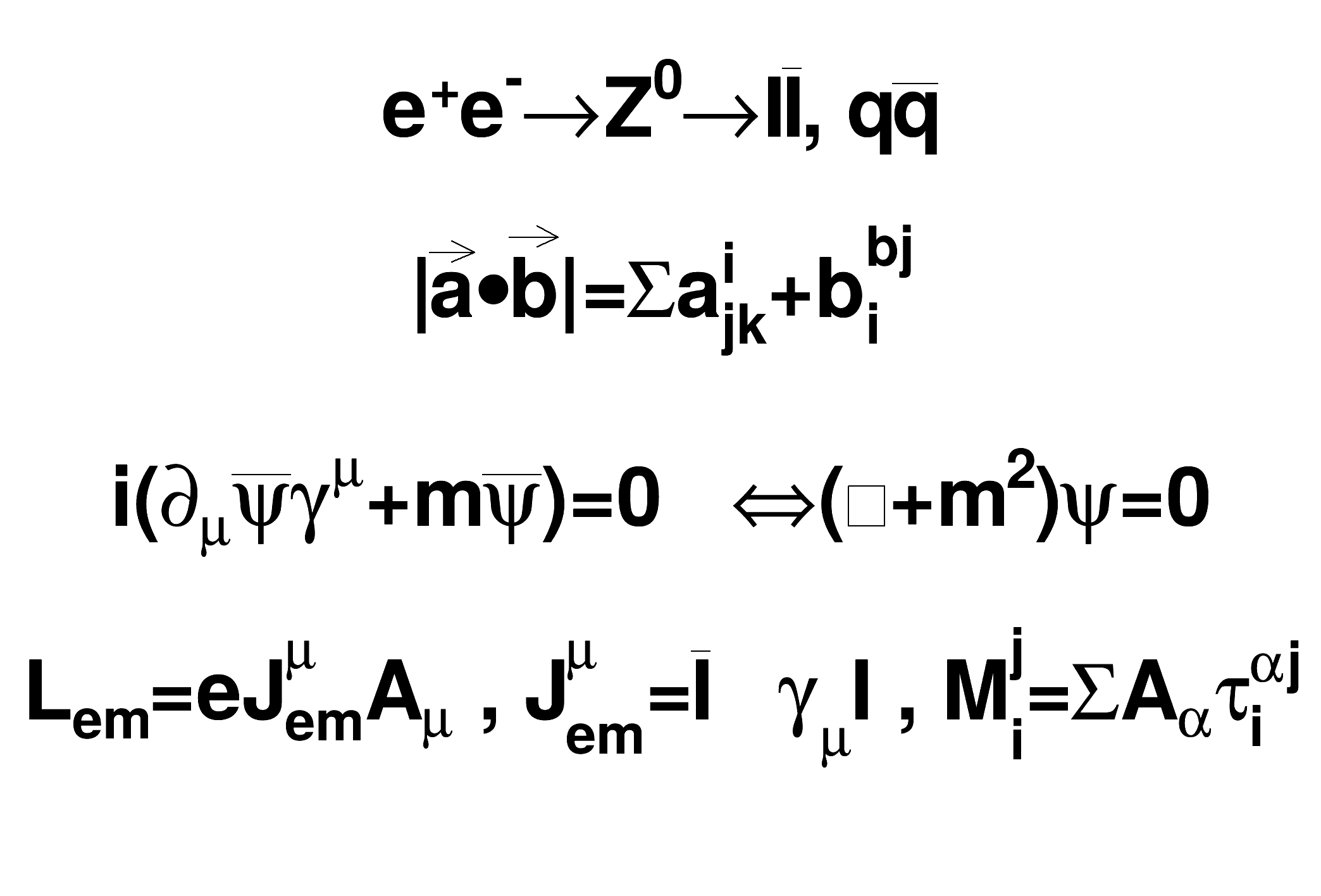ROOT   Reference Guide
Searching...
No Matches
latex2.C File Reference

## Detailed DescriptionThis macro draws 4 Latex-style formula in a canvas and prints the canvas as a Postscript file.void latex2(){
TCanvas *c1 = new TCanvas("c1");
l.SetTextAlign(23);
l.SetTextSize(0.1);
l.DrawLatex(0.5,0.95,"e^{+}e^{-}#rightarrowZ^{0}#rightarrowI#bar{I}, q#bar{q}");
l.DrawLatex(0.5,0.75,"|#vec{a}#bullet#vec{b}|=#Sigmaa^{i}_{jk}+b^{bj}_{i}");
l.DrawLatex(0.5,0.5,"i(#partial_{#mu}#bar{#psi}#gamma^{#mu}+m#bar{#psi})=0\
#Leftrightarrow(#Box+m^{2})#psi=0");
l.DrawLatex(0.5,0.3,"L_{em}=eJ^{#mu}_{em}A_{#mu} , J^{#mu}_{em}=#bar{I}\
#gamma_{#mu}I , M^{j}_{i}=#SigmaA_{#alpha}#tau^{#alphaj}_{i}");
c1->Print("latex2.ps");
}
The Canvas class.
Definition TCanvas.h:23
To draw Mathematical Formula.
Definition TLatex.h:18
void Print(Option_t *option="") const override
Dump this line with its attributes.
Definition TLine.cxx:419
return c1
Definition legend1.C:41
TLine l
Definition textangle.C:4

Definition in file latex2.C.